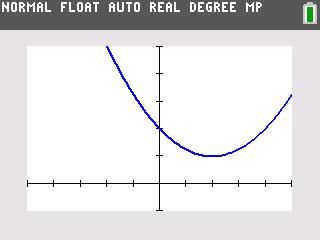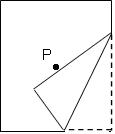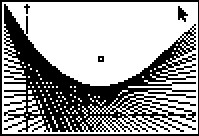••• ##### Device
• TI-83 Plus Family
• TI-84 Plus
• TI-84 Plus Silver Edition
•TI-84 Plus C Silver Edition
•TI-84 Plus CE

# Precalculus: Exploring the Parabola

by Texas Instruments#### Overview

Students explore the key features of the parabola, both geometrically and algebraically.

#### Key Steps

•In problem 1, students will use a sheet of paper to explore parabolas. They will start by marking a point on the center of the paper and producing a series of folds along one of the longer edges that pass through this point.

•Tracing along these straight-line folds should produce an envelope of lines, and the locus formed by the paper folding gives and approximation of a parabola. Students may use Cabri® Jr. to do a similar exploration.

Students move on to fitting a parabola, they use the formula 4p(y - k) = (x - h)2 to assist them in their exploration.

•At the end of this activity, students will be able to write and graph an equation of a parabola with vertex at (h, k) and axis of symmetry x = h or y = k. Students will also be able to derive the formula for any conic.